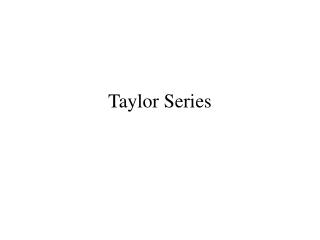DownloadDownload PresentationTaylor Series

# Taylor Series

Télécharger la présentation## Taylor Series

- - - - - - - - - - - - - - - - - - - - - - - - - - - E N D - - - - - - - - - - - - - - - - - - - - - - - - - - -
##### Presentation Transcript

1. Taylor Series

2. SOLUTION OFNON-LINEAR EQUATIONS • All equations used in horizontal adjustment are non-linear. • Solution involves approximating solution using 1'st order Taylor series expansion, and • Then solving system for corrections to approximate solution. • Repeat solving system of linearized equations for corrections until corrections become small. • This process of solving equations is known as: ITERATING

3. Taylor’s Series Given a function, L = f(x,y)

4. Taylor’s Series • The series is also non-linear (unknowns are the dx’s, dy’s, and higher order terms) • Therefore, truncate the series after only the first order terms, which makes the equation an approximation • Initial approximations generally need to be reasonably close in order for the solution to converge

5. Solution • Determine initial approximations (closer is better) • Form the (first order) equations • Solve for corrections, dx and dy • Add corrections to approximations to get improved values • Iterate until the solution converges

6. Example C.1 Solve the following pair of non-linear equations. Use initial approximation of 1 (one) for both x and y. First, determine the partial derivatives

7. Partials

8. Write the Linearized Equations Simplify

9. Solve New approximations:

10. Solve – Iteration 2 New approximations:

11. Iteration 3 Same procedure yields: dx = 0.00 and dy = -0.11 This results in new approximations of x = 2.00 and y = 2.00 Further iterations are negligible

12. General Matrix Form • The coefficient matrix formed by the partial derivatives of the functions with respect to the variables is the Jacobian matrix • It can be used directly in a general matrix form

13. General Form for Example JX = K

14. Circle Example Determine the equation of a circle that passes through the points (9.4, 5.6), (7.6, 7.2), and (3.8, 4.8). Initial approximations for unknown and circle equation: Center point: (7, 4.5), Radius: 3 Rearranged Linearizing

15. Set Up General Matrix Form

16. Substitute the Values and Solve

17. Continue Until Converged